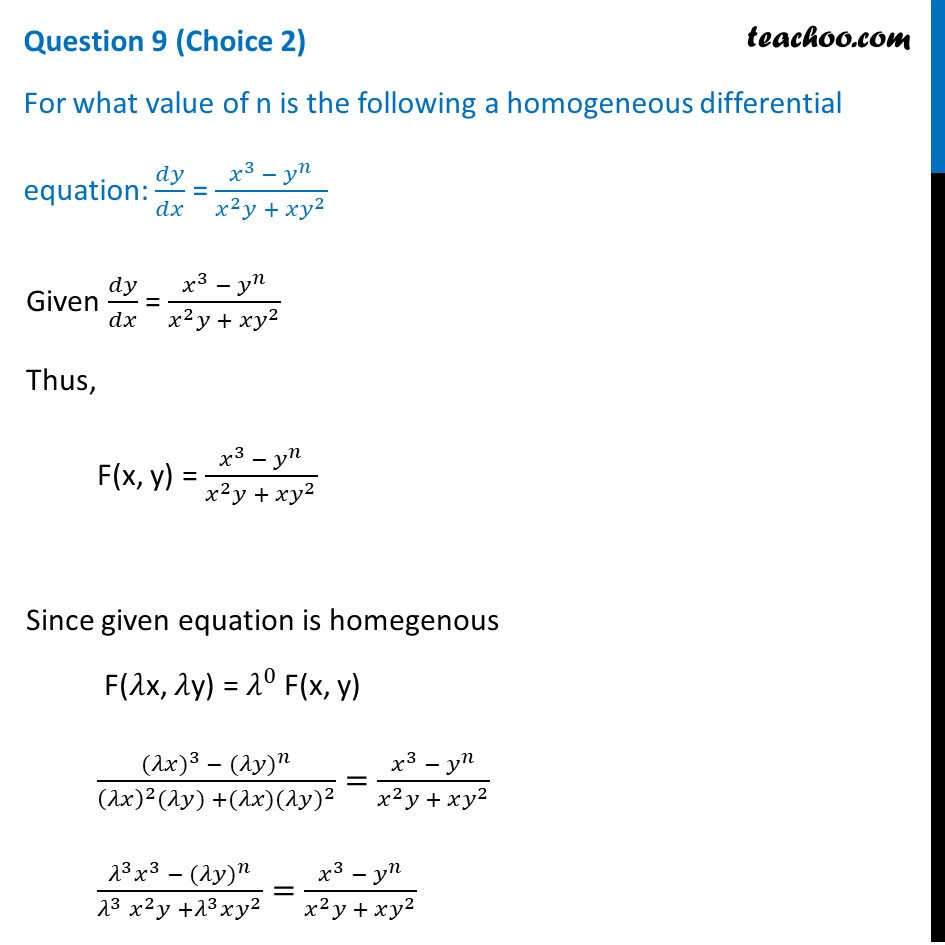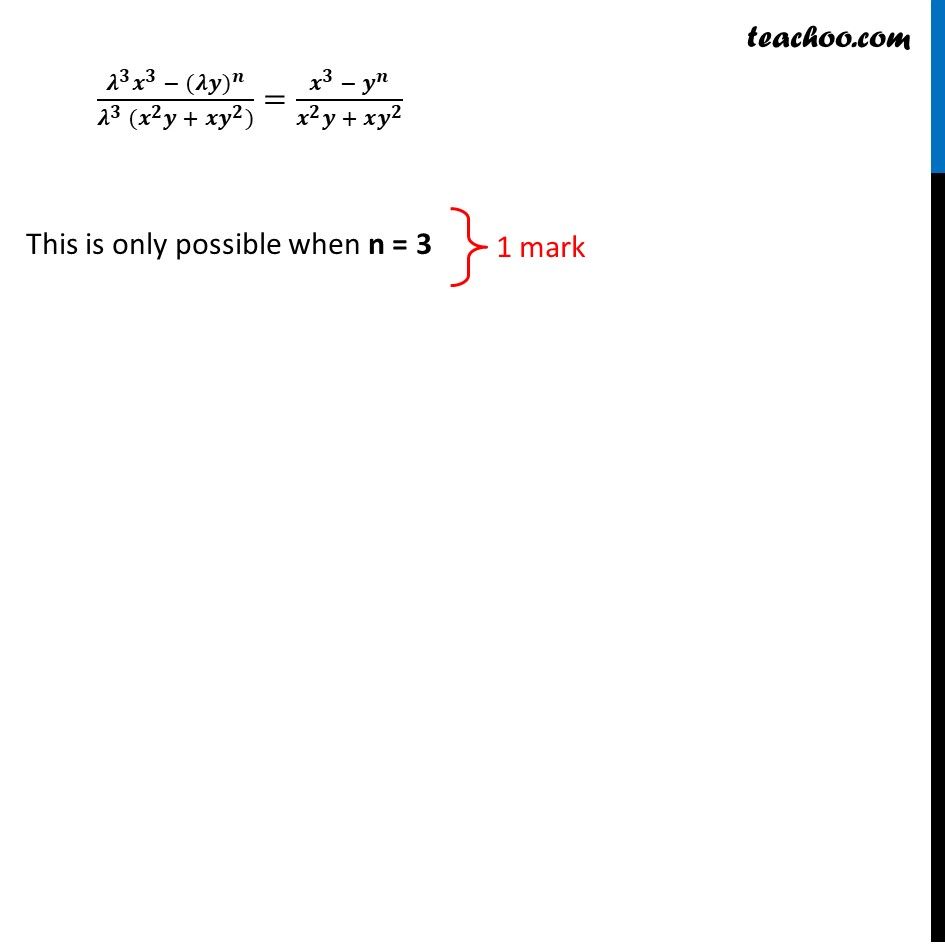## For what value of n is the following a homogeneous differential equation: dy/dx = x 3 - yn / x 2 y + xy 21. Class 12
2. Solutions of Sample Papers and Past Year Papers - for Class 12 Boards
3. CBSE Class 12 Sample Paper for 2021 Boards

Transcript

Question 9 (Choice 2) For what value of n is the following a homogeneous differential equation: 𝑑𝑦/𝑑𝑥 = (𝑥^3 − 𝑦^𝑛)/(𝑥^2 𝑦 + 𝑥𝑦^2 ) Given 𝑑𝑦/𝑑𝑥 = (𝑥^3 − 𝑦^𝑛)/(𝑥^2 𝑦 + 𝑥𝑦^2 ) Thus, F(x, y) = (𝑥^3 − 𝑦^𝑛)/(𝑥^2 𝑦 + 𝑥𝑦^2 ) Since given equation is homegenous F(𝜆x, 𝜆y) = 𝜆^0 F(x, y) (〖(𝜆𝑥)〗^3 −〖 (𝜆𝑦)〗^𝑛)/((𝜆𝑥)^2 (𝜆𝑦) +(𝜆𝑥)〖(𝜆𝑦)〗^2 )=(𝑥^3 − 𝑦^𝑛)/(𝑥^2 𝑦 + 𝑥𝑦^2 ) (𝜆^3 𝑥^3 − 〖(𝜆𝑦)〗^𝑛)/(𝜆^3 𝑥^2 𝑦 +𝜆^3 𝑥𝑦^2 )=(𝑥^3 − 𝑦^𝑛)/(𝑥^2 𝑦 + 𝑥𝑦^2 ) (𝝀^𝟑 𝒙^𝟑 − 〖(𝝀𝒚)〗^𝒏)/(𝝀^𝟑 (𝒙^𝟐 𝒚 + 𝒙𝒚^𝟐))=(𝒙^𝟑 − 𝒚^𝒏)/(𝒙^𝟐 𝒚 + 𝒙𝒚^𝟐 ) This is only possible when n = 3

CBSE Class 12 Sample Paper for 2021 Boards

Class 12
Solutions of Sample Papers and Past Year Papers - for Class 12 Boards